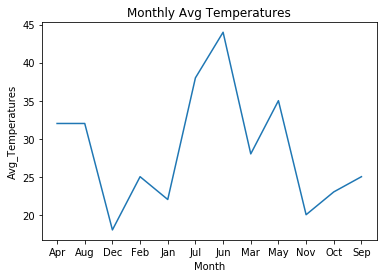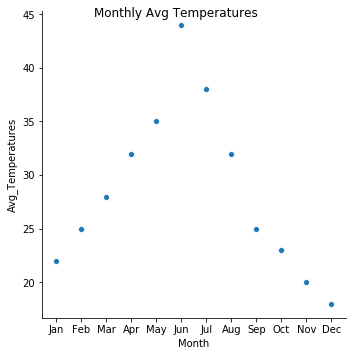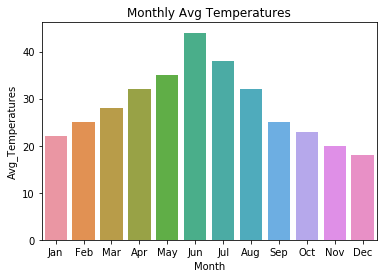# How to Add a Title to Seaborn Plots?

• Last Updated : 19 Dec, 2021

## Method 1: Adding title using set method

set method takes 1 argument “title” as a parameter which stores Title of a plot.

Syntax

set(title=”Title of a plot”)

Example:

Here, we are going to create a dataframe with months and average temperatures

## Python3

 `# import necessary packages ` `import` `pandas as pd ` `import` `seaborn as sns ` ` `  `# create a dataframe ` `temperature ``=` `pd.DataFrame({``'Month'``: [``'Jan'``, ``'Feb'``, ``'Mar'``, ` `                                      ``'Apr'``, ``'May'``, ``'Jun'``,  ` `                                      ``'Jul'``, ``'Aug'``, ``'Sep'``, ` `                                      ``'Oct'``, ``'Nov'``, ``'Dec'``], ` `                            ``'Avg_Temperatures'``: [``22``, ``25``, ``28``, ` `                                                 ``32``, ``35``, ``44``,  ` `                                                 ``38``, ``32``, ``25``,  ` `                                                 ``23``, ``20``, ``18``]}) ` ` `  `# plot a line plot and set title ` `sns.lineplot(x``=``'Month'``, y``=``'Avg_Temperatures'``, data``=``temperature).``set``( ` `    ``title``=``"Monthly Avg Temperatures"``) `

Output## Method 2: Adding title using suptitle method

suptitle method takes a string which is title of plot as parameter.

Syntax

fig.suptitle(‘Title of plot’)

Example:

## Python3

 `# import necessary packages ` `import` `pandas as pd ` `import` `seaborn as sns ` ` `  `# create a dataframe ` `temperature ``=` `pd.DataFrame({``'Month'``: [``'Jan'``, ``'Feb'``, ``'Mar'``, ` `                                      ``'Apr'``, ``'May'``, ``'Jun'``, ` `                                      ``'Jul'``, ``'Aug'``, ``'Sep'``, ` `                                      ``'Oct'``, ``'Nov'``, ``'Dec'``], ` `                            ``'Avg_Temperatures'``: [``22``, ``25``, ``28``, ` `                                                 ``32``, ``35``, ``44``,  ` `                                                 ``38``, ``32``, ``25``,  ` `                                                 ``23``, ``20``, ``18``]}) ` ` `  `# plot a rel-plot ` `plot ``=` `sns.relplot(x``=``'Month'``, y``=``'Avg_Temperatures'``, data``=``temperature) ` ` `  `# add title using suptitle ` `plot.fig.suptitle(``'Monthly Avg Temperatures'``) `

Output## Method 3: Adding title using set_title

set_title method takes a string as parameter which is title of plot.

Syntax

set_title(‘Title of plot’)

Example:

## Python3

 `# import necessary packages ` `import` `pandas as pd ` `import` `seaborn as sns ` ` `  `# create a dataframe ` `temperature ``=` `pd.DataFrame({``'Month'``: [``'Jan'``, ``'Feb'``, ``'Mar'``,  ` `                                      ``'Apr'``, ``'May'``, ``'Jun'``,  ` `                                      ``'Jul'``, ``'Aug'``, ``'Sep'``, ` `                                      ``'Oct'``, ``'Nov'``, ``'Dec'``], ` `                            ``'Avg_Temperatures'``: [``22``, ``25``, ``28``, ` `                                                 ``32``, ``35``, ``44``,  ` `                                                 ``38``, ``32``, ``25``, ` `                                                 ``23``, ``20``, ``18``]}) ` ` `  `# plot a bar plot and add title using set_title ` `plot ``=` `sns.barplot(x``=``'Month'``, y``=``'Avg_Temperatures'``, ` `                   ``data``=``temperature).set_title(``'Monthly Avg Temperatures'``) `

OutputMy Personal Notes arrow_drop_up
Recommended Articles
Page :